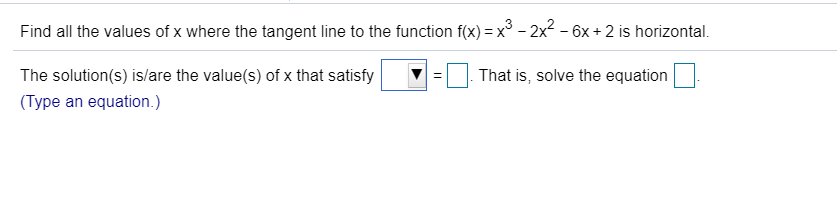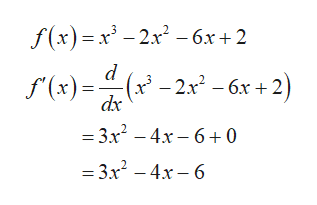Find all the values of x where the tangent line to the function f(x) x3 - 2x2 - 6x + 2 is horizontal.That is, solve the equationThe solution(s) is/are the value(s) of x that satisfy(Type an equation.)

Questionhelp_outlineImage TranscriptioncloseFind all the values of x where the tangent line to the function f(x) x3 - 2x2 - 6x + 2 is horizontal. That is, solve the equation The solution(s) is/are the value(s) of x that satisfy (Type an equation.) fullscreen
Step 1

First compute the deriva...help_outlineImage Transcriptionclosef(x)=x3 -2x2-6x+2 d f'(x)=(x'-2.x2 - 6x+; dx = 3x2 -4x- 60 = 3x2 -4x- 6 fullscreen

Want to see the full answer?

See Solution

Want to see this answer and more?

Our solutions are written by experts, many with advanced degrees, and available 24/7

See Solution
Tagged in

Derivative